# gptools package¶

## gptools.error_handling module¶

Contains exceptions specific to the gptools package.

exception gptools.error_handling.GPArgumentError[source]

Bases: exceptions.Exception

Exception class raised when an incorrect combination of keyword arguments is given.

exception gptools.error_handling.GPImpossibleParamsError[source]

Bases: exceptions.Exception

Exception class raised when parameters are not possible.

## gptools.gaussian_process module¶

Provides the base GaussianProcess class.

class gptools.gaussian_process.GaussianProcess(k, noise_k=None, X=None, y=None, err_y=0, n=0, T=None, diag_factor=100.0, mu=None, use_hyper_deriv=False, verbose=False)[source]

Bases: object

Gaussian process.

If called with one argument, an untrained Gaussian process is constructed and data must be added with the add_data() method. If called with the optional keywords, the values given are used as the data. It is always possible to add additional data with add_data().

Note that the attributes have no write protection, but you should always add data with add_data() to ensure internal consistency.

Parameters: k : Kernel instance Kernel instance corresponding to the desired noise-free covariance kernel of the Gaussian process. The noise is handled separately either through specification of err_y, or in a separate kernel. This allows noise-free predictions when needed. noise_k : Kernel instance Kernel instance corresponding to the noise portion of the desired covariance kernel of the Gaussian process. Note that you DO NOT need to specify this if the extent of the noise you want to represent is contained in err_y (or if your data are noiseless). Default value is None, which results in the ZeroKernel (noise specified elsewhere or not present). diag_factor : float, optional Factor of sys.float_info.epsilon which is added to the diagonal of the total K matrix to improve the stability of the Cholesky decomposition. If you are having issues, try increasing this by a factor of 10 at a time. Default is 1e2. mu : MeanFunction instance The mean function of the Gaussian process. Default is None (zero mean prior). NOTE The following are all passed to add_data(), refer to its docstring. X : array, (N, D), optional M input values of dimension D. Default value is None (no data). y : array, (M,), optional M data target values. Default value is None (no data). err_y : array, (M,), optional Error (given as standard deviation) in the M training target values. Default value is 0 (noiseless observations). n : array, (N, D) or scalar float, optional Non-negative integer values only. Degree of derivative for each target. If n is a scalar it is taken to be the value for all points in y. Otherwise, the length of n must equal the length of y. Default value is 0 (observation of target value). If non-integer values are passed, they will be silently rounded. T : array, (M, N), optional Linear transformation to get from latent variables to data in the argument y. When T is passed the argument y holds the transformed quantities y=TY(X) where y are the observed values of the transformed quantities, T is the transformation matrix and Y(X) is the underlying (untransformed) values of the function to be fit that enter into the transformation. When T is M-by-N and y has M elements, X and n will both be N-by-D. Default is None (no transformation). use_hyper_deriv : bool, optional If True, the elements needed to compute the derivatives of the log-likelihood with respect to the hyperparameters will be computed. Default is False (do not compute these elements). Derivatives with respect to hyperparameters are currently only supported for the squared exponential covariance kernel. verbose : bool, optional If True, warnings which are produced internally but are not critical will not be generated. This does not control when a warning happens in a routine which is called, however. Default is False (do not produce warnings). TypeError k or noise_k is not an instance of Kernel. GPArgumentError Gave X but not y (or vice versa). ValueError Training data rejected by add_data().

add_data
Used to process X, y, err_y and to add data.

Attributes

 num_dim The number of dimensions of the input data. hyperprior Combined hyperprior for the kernel, noise kernel and (if present) mean function. params Combined hyperparameters for the kernel, noise kernel and (if present) mean function. param_bounds Combined bounds for the hyperparameters for the kernel, noise kernel and (if present) mean function. param_names Combined names for the hyperparameters for the kernel, noise kernel and (if present) mean function. fixed_params Combined fixed hyperparameter flags for the kernel, noise kernel and (if present) mean function. free_params Combined free hyperparameters for the kernel, noise kernel and (if present) mean function. free_param_bounds Combined free hyperparameter bounds for the kernel, noise kernel and (if present) mean function. free_param_names Combined free hyperparameter names for the kernel, noise kernel and (if present) mean function.
 k (Kernel instance) The non-noise portion of the covariance kernel. noise_k (Kernel instance) The noise portion of the covariance kernel. mu (MeanFunction instance) Parametric mean function. X (array, (M, D)) The M training input values, each of which is of dimension D. n (array, (M, D)) The orders of derivatives that each of the M training points represent, indicating the order of derivative with respect to each of the D dimensions. T (array, (M, N)) The transformation matrix applied to the training data. If this is not None, X and n will be N-by-D. y (array, (M,)) The M training target values. err_y (array, (M,)) The uncorrelated, possibly heteroscedastic uncertainty in the M training input values. K (array, (M, M)) Covariance matrix between all of the training inputs. noise_K (array, (M, M)) Noise portion of the covariance matrix between all of the training inputs. Note that if T is present this is applied between all of the training quadrature points, so additional noise on the transformed observations cannot be inferred. L (array, (M, M)) Lower-triangular Cholesky decomposition of the total covariance matrix between all of the training inputs. alpha (array, (M, 1)) Solution to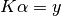. ll (float) Log-posterior density of the model. diag_factor (float) The factor of sys.float_info.epsilon which is added to the diagonal of the K matrix to improve stability. K_up_to_date (bool) True if no data have been added since the last time the internal state was updated with a call to compute_K_L_alpha_ll(). use_hyper_deriv (bool) Whether or not the derivative of the log-posterior with respect to the hyperparameters should be computed/used. verbose (bool) Whether or not to print non-critical, internally-generated warnings.
hyperprior

Combined hyperprior for the kernel, noise kernel and (if present) mean function.

fixed_params

Combined fixed hyperparameter flags for the kernel, noise kernel and (if present) mean function.

params

Combined hyperparameters for the kernel, noise kernel and (if present) mean function.

param_bounds

Combined bounds for the hyperparameters for the kernel, noise kernel and (if present) mean function.

param_names

Combined names for the hyperparameters for the kernel, noise kernel and (if present) mean function.

free_params

Combined free hyperparameters for the kernel, noise kernel and (if present) mean function.

free_param_bounds

Combined free hyperparameter bounds for the kernel, noise kernel and (if present) mean function.

free_param_names

Combined free hyperparameter names for the kernel, noise kernel and (if present) mean function.

add_data(X, y, err_y=0, n=0, T=None)[source]

Add data to the training data set of the GaussianProcess instance.

Parameters: X : array, (M, D) M input values of dimension D. y : array, (M,) M target values. err_y : array, (M,) or scalar float, optional Non-negative values only. Error given as standard deviation) in the M target values. If err_y is a scalar, the data set is taken to be homoscedastic (constant error). Otherwise, the length of err_y must equal the length of y. Default value is 0 (noiseless observations). n : array, (M, D) or scalar float, optional Non-negative integer values only. Degree of derivative for each target. If n is a scalar it is taken to be the value for all points in y. Otherwise, the length of n must equal the length of y. Default value is 0 (observation of target value). If non-integer values are passed, they will be silently rounded. T : array, (M, N), optional Linear transformation to get from latent variables to data in the argument y. When T is passed the argument y holds the transformed quantities y=TY(X) where y are the observed values of the transformed quantities, T is the transformation matrix and Y(X) is the underlying (untransformed) values of the function to be fit that enter into the transformation. When T is M-by-N and y has M elements, X and n will both be N-by-D. Default is None (no transformation). ValueError Bad shapes for any of the inputs, negative values for err_y or n.
condense_duplicates()[source]

Condense duplicate points using a transformation matrix.

This is useful if you have multiple non-transformed points at the same location or multiple transformed points that use the same quadrature points.

Won’t change the GP if all of the rows of [X, n] are unique. Will create a transformation matrix T if necessary. Note that the order of the points in [X, n] will be arbitrary after this operation.

If there are any transformed quantities (i.e., self.T is not None), it will also remove any quadrature points for which all of the weights are zero (even if all of the rows of [X, n] are unique).

remove_outliers(thresh=3, **predict_kwargs)[source]

Remove outliers from the GP with very simplistic outlier detection.

Removes points that are more than thresh * err_y away from the GP mean. Note that this is only very rough in that it ignores the uncertainty in the GP mean at any given point. But you should only be using this as a rough way of removing bad channels, anyways!

Returns the values that were removed and a boolean array indicating where the removed points were.

Parameters: thresh : float, optional The threshold as a multiplier times err_y. Default is 3 (i.e., throw away all 3-sigma points). **predict_kwargs : optional kwargs All additional kwargs are passed to predict(). You can, for instance, use this to make it use MCMC to evaluate the mean. (If you don’t use MCMC, then the current value of the hyperparameters is used.) X_bad : array Input values of the bad points. y_bad : array Bad values. err_y_bad : array Uncertainties on the bad values. n_bad : array Derivative order of the bad values. bad_idxs : array Array of booleans with the original shape of X with True wherever a point was taken to be bad and subsequently removed. T_bad : array Transformation matrix of returned points. Only returned if T is not None for the instance.
optimize_hyperparameters(method='SLSQP', opt_kwargs={}, verbose=False, random_starts=None, num_proc=None, max_tries=1)[source]

Optimize the hyperparameters by maximizing the log-posterior.

Leaves the GaussianProcess instance in the optimized state.

If scipy.optimize.minimize() is not available (i.e., if your scipy version is older than 0.11.0) then fmin_slsqp() is used independent of what you set for the method keyword.

If use_hyper_deriv is True the optimizer will attempt to use the derivatives of the log-posterior with respect to the hyperparameters to speed up the optimization. Note that only the squared exponential covariance kernel supports hyperparameter derivatives at present.

Parameters: method : str, optional The method to pass to scipy.optimize.minimize(). Refer to that function’s docstring for valid options. Default is ‘SLSQP’. See note above about behavior with older versions of scipy. opt_kwargs : dict, optional Dictionary of extra keywords to pass to scipy.optimize.minimize(). Refer to that function’s docstring for valid options. Default is: {}. verbose : bool, optional Whether or not the output should be verbose. If True, the entire Result object from scipy.optimize.minimize() is printed. If False, status information is only printed if the success flag from minimize() is False. Default is False. random_starts : non-negative int, optional Number of times to randomly perturb the starting guesses (distributed according to the hyperprior) in order to seek the global minimum. If None, then num_proc random starts will be performed. Default is None (do number of random starts equal to the number of processors allocated). Note that for random_starts != 0, the initial state of the hyperparameters is not actually used. num_proc : non-negative int or None, optional Number of processors to use with random starts. If 0, processing is not done in parallel. If None, all available processors are used. Default is None (use all available processors). max_tries : int, optional Number of times to run through the random start procedure if a solution is not found. Default is to only go through the procedure once.
predict(Xstar, n=0, noise=False, return_std=True, return_cov=False, full_output=False, return_samples=False, num_samples=1, samp_kwargs={}, return_mean_func=False, use_MCMC=False, full_MC=False, rejection_func=None, ddof=1, output_transform=None, **kwargs)[source]

Predict the mean and covariance at the inputs Xstar.

The order of the derivative is given by n. The keyword noise sets whether or not noise is included in the prediction.

Parameters:

Xstar : array, (M, D)

M test input values of dimension D.

n : array, (M, D) or scalar, non-negative int, optional

Order of derivative to predict (0 is the base quantity). If n is scalar, the value is used for all points in Xstar. If non-integer values are passed, they will be silently rounded. Default is 0 (return base quantity).

noise : bool, optional

Whether or not noise should be included in the covariance. Default is False (no noise in covariance).

return_std : bool, optional

Set to True to compute and return the standard deviation for the predictions, False to skip this step. Default is True (return tuple of (mean, std)).

return_cov : bool, optional

Set to True to compute and return the full covariance matrix for the predictions. This overrides the return_std keyword. If you want both the standard deviation and covariance matrix pre-computed, use the full_output keyword.

full_output : bool, optional

Set to True to return the full outputs in a dictionary with keys:

 mean mean of GP at requested points std standard deviation of GP at requested points cov covariance matrix for values of GP at requested points samp random samples of GP at requested points (only if return_samples is True) mean_func mean function of GP (only if return_mean_func is True) cov_func covariance of mean function of GP (zero if not using MCMC) std_func standard deviation of mean function of GP (zero if not using MCMC) mean_without_func mean of GP minus mean function of GP cov_without_func covariance matrix of just the GP portion of the fit std_without_func standard deviation of just the GP portion of the fit

return_samples : bool, optional

Set to True to compute and return samples of the GP in addition to computing the mean. Only done if full_output is True. Default is False.

num_samples : int, optional

Number of samples to compute. If using MCMC this is the number of samples per MCMC sample, if using present values of hyperparameters this is the number of samples actually returned. Default is 1.

samp_kwargs : dict, optional

Additional keywords to pass to draw_sample() if return_samples is True. Default is {}.

return_mean_func : bool, optional

Set to True to return the evaluation of the mean function in addition to computing the mean of the process itself. Only done if full_output is True and self.mu is not None. Default is False.

use_MCMC : bool, optional

Set to True to use predict_MCMC() to evaluate the prediction marginalized over the hyperparameters.

full_MC : bool, optional

Set to True to compute the mean and covariance matrix using Monte Carlo sampling of the posterior. The samples will also be returned if full_output is True. The sample mean and covariance will be evaluated after filtering through rejection_func, so conditional means and covariances can be computed. Default is False (do not use full sampling).

rejection_func : callable, optional

Any samples where this function evaluates False will be rejected, where it evaluates True they will be kept. Default is None (no rejection). Only has an effect if full_MC is True.

ddof : int, optional

The degree of freedom correction to use when computing the covariance matrix when full_MC is True. Default is 1 (unbiased estimator).

output_transform: array, (L, M), optional

Matrix to use to transform the output vector of length M to one of length L. This can, for instance, be used to compute integrals.

**kwargs : optional kwargs

All additional kwargs are passed to predict_MCMC() if use_MCMC is True.

Returns:

mean : array, (M,)

Predicted GP mean. Only returned if full_output is False.

std : array, (M,)

Predicted standard deviation, only returned if return_std is True, return_cov is False and full_output is False.

cov : array, (M, M)

Predicted covariance matrix, only returned if return_cov is True and full_output is False.

full_output : dict

Dictionary with fields for mean, std, cov and possibly random samples and the mean function. Only returned if full_output is True.

Raises:

ValueError

If n is not consistent with the shape of Xstar or is not entirely composed of non-negative integers.

plot(X=None, n=0, ax=None, envelopes=[1, 3], base_alpha=0.375, return_prediction=False, return_std=True, full_output=False, plot_kwargs={}, **kwargs)[source]

Plots the Gaussian process using the current hyperparameters. Only for num_dim <= 2.

Parameters:

X : array-like (M,) or (M, num_dim), optional

The values to evaluate the Gaussian process at. If None, then 100 points between the minimum and maximum of the data’s X are used for a univariate Gaussian process and a 50x50 grid is used for a bivariate Gaussian process. Default is None (use 100 points between min and max).

n : int or list, optional

The order of derivative to compute. For num_dim=1, this must be an int. For num_dim=2, this must be a list of ints of length 2. Default is 0 (don’t take derivative).

ax : axis instance, optional

Axis to plot the result on. If no axis is passed, one is created. If the string ‘gca’ is passed, the current axis (from plt.gca()) is used. If X_dim = 2, the axis must be 3d.

envelopes: list of float, optional

+/-n*sigma envelopes to plot. Default is [1, 3].

base_alpha : float, optional

Alpha value to use for +/-1*sigma envelope. All other envelopes env are drawn with base_alpha/env. Default is 0.375.

return_prediction : bool, optional

If True, the predicted values are also returned. Default is False.

return_std : bool, optional

If True, the standard deviation is computed and returned along with the mean when return_prediction is True. Default is True.

full_output : bool, optional

Set to True to return the full outputs in a dictionary with keys:

 mean mean of GP at requested points std standard deviation of GP at requested points cov covariance matrix for values of GP at requested points samp random samples of GP at requested points (only if return_sample is True)

plot_kwargs : dict, optional

The entries in this dictionary are passed as kwargs to the plotting command used to plot the mean. Use this to, for instance, change the color, line width and line style.

**kwargs : extra arguments for predict, optional

Extra arguments that are passed to predict().

Returns:

ax : axis instance

The axis instance used.

mean : Array, (M,)

Predicted GP mean. Only returned if return_prediction is True and full_output is False.

std : Array, (M,)

Predicted standard deviation, only returned if return_prediction and return_std are True and full_output is False.

full_output : dict

Dictionary with fields for mean, std, cov and possibly random samples. Only returned if return_prediction and full_output are True.

draw_sample(Xstar, n=0, num_samp=1, rand_vars=None, rand_type='standard normal', diag_factor=1000.0, method='cholesky', num_eig=None, mean=None, cov=None, modify_sign=None, **kwargs)[source]

Draw a sample evaluated at the given points Xstar.

Note that this function draws samples from the GP given the current values for the hyperparameters (which may be in a nonsense state if you just created the instance or called a method that performs MCMC sampling). If you want to draw random samples from MCMC output, use the return_samples and full_output keywords to predict().

Parameters: Xstar : array, (M, D) M test input values of dimension D. n : array, (M, D) or scalar, non-negative int, optional Derivative order to evaluate at. Default is 0 (evaluate value). noise : bool, optional Whether or not to include the noise components of the kernel in the sample. Default is False (no noise in samples). num_samp : Positive int, optional Number of samples to draw. Default is 1. Cannot be used in conjunction with rand_vars: If you pass both num_samp and rand_vars, num_samp will be silently ignored. rand_vars : array, (M, P), optional Vector of random variables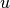to use in constructing the sample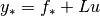, where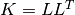. If None, values will be produced using numpy.random.multivariate_normal(). This allows you to use pseudo/quasi random numbers generated by an external routine. Note that, when method is ‘eig’, the eigenvalues are in ascending order. Default is None (use multivariate_normal() directly). rand_type : {‘standard normal’, ‘uniform’}, optional Type of distribution the inputs are given with. ‘standard normal’: Standard (mu = 0, sigma = 1) normal distribution (this is the default) ‘uniform’: Uniform distribution on [0, 1). In this case the required Gaussian variables are produced with inversion. diag_factor : float, optional Number (times machine epsilon) added to the diagonal of the covariance matrix prior to computing its Cholesky decomposition. This is necessary as sometimes the decomposition will fail because, to machine precision, the matrix appears to not be positive definite. If you are getting errors from scipy.linalg.cholesky(), try increasing this an order of magnitude at a time. This parameter only has an effect when using rand_vars. Default value is 1e3. method : {‘cholesky’, ‘eig’}, optional Method to use for constructing the matrix square root. Default is ‘cholesky’ (use lower-triangular Cholesky decomposition). ‘cholesky’: Perform Cholesky decomposition on the covariance matrix:, use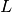as the matrix square root. ‘eig’: Perform an eigenvalue decomposition on the covariance matrix: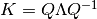, use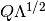as the matrix square root. num_eig : int or None, optional Number of eigenvalues to compute. Can range from 1 to M (the number of test points). If it is None, then all eigenvalues are computed. Default is None (compute all eigenvalues). This keyword only has an effect if method is ‘eig’. mean : array, (M,), optional If you have pre-computed the mean and covariance matrix, then you can simply pass them in with the mean and cov keywords to save on having to call predict(). cov : array, (M, M), optional If you have pre-computed the mean and covariance matrix, then you can simply pass them in with the mean and cov keywords to save on having to call predict(). modify_sign : {None, ‘left value’, ‘right value’, ‘left slope’, ‘right slope’, ‘left concavity’, ‘right concavity’}, optional If None (the default), the eigenvectors as returned by scipy.linalg.eigh() are used without modification. To modify the sign of the eigenvectors (necessary for some advanced use cases), set this kwarg to one of the following: ‘left value’: forces the first value of each eigenvector to be positive. ‘right value’: forces the last value of each eigenvector to be positive. ‘left slope’: forces the slope to be positive at the start of each eigenvector. ‘right slope’: forces the slope to be positive at the end of each eigenvector. ‘left concavity’: forces the second derivative to be positive at the start of each eigenvector. ‘right concavity’: forces the second derivative to be positive at the end of each eigenvector. **kwargs : optional kwargs All extra keyword arguments are passed to predict() when evaluating the mean and covariance matrix of the GP. samples : Array (M, P) or (M, num_samp) Samples evaluated at the M points. ValueError If rand_type or method is invalid.
update_hyperparameters(new_params, hyper_deriv_handling='default', exit_on_bounds=True, inf_on_error=True)[source]

Update the kernel’s hyperparameters to the new parameters.

This will call compute_K_L_alpha_ll() to update the state accordingly.

Note that if this method crashes and the hyper_deriv_handling keyword was used, it may leave use_hyper_deriv in the wrong state.

Parameters: new_params : Array or other Array-like, length dictated by kernel New parameters to use. hyper_deriv_handling : {‘default’, ‘value’, ‘deriv’}, optional Determines what to compute and return. If ‘default’ and use_hyper_deriv is True then the negative log-posterior and the negative gradient of the log-posterior with respect to the hyperparameters is returned. If ‘default’ and use_hyper_deriv is False or ‘value’ then only the negative log-posterior is returned. If ‘deriv’ then only the negative gradient of the log-posterior with respect to the hyperparameters is returned. exit_on_bounds : bool, optional If True, the method will automatically exit if the hyperparameters are impossible given the hyperprior, without trying to update the internal state. This is useful during MCMC sampling and optimization. Default is True (don’t perform update for impossible hyperparameters). inf_on_error : bool, optional If True, the method will return scipy.inf if the hyperparameters produce a linear algebra error upon trying to update the Gaussian process. Default is True (catch errors and return infinity). -1*ll : float The updated log posterior. -1*ll_deriv : array of float, (num_params,) The gradient of the log posterior. Only returned if use_hyper_deriv is True or hyper_deriv_handling is set to ‘deriv’.
compute_K_L_alpha_ll()[source]

Compute K, L, alpha and log-likelihood according to the first part of Algorithm 2.1 in R&W.

Computes K and the noise portion of K using compute_Kij(), computes L using scipy.linalg.cholesky(), then computes alpha as L.T\(L\y).

Only does the computation if K_up_to_date is False – otherwise leaves the existing values.

num_dim

The number of dimensions of the input data.

Returns: num_dim: int The number of dimensions of the input data as defined in the kernel.
compute_Kij(Xi, Xj, ni, nj, noise=False, hyper_deriv=None, k=None)[source]

Compute covariance matrix between datasets Xi and Xj.

Specify the orders of derivatives at each location with the ni, nj arrays. The include_noise flag is passed to the covariance kernel to indicate whether noise is to be included (i.e., for evaluation of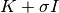versus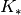).

If Xj is None, the symmetric matrix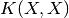is formed.

Note that type and dimension checking is NOT performed, as it is assumed the data are from inside the instance and have hence been sanitized by add_data().

Parameters: Xi : array, (M, D) M input values of dimension D. Xj : array, (P, D) P input values of dimension D. ni : array, (M, D), non-negative integers M derivative orders with respect to the Xi coordinates. nj : array, (P, D), non-negative integers P derivative orders with respect to the Xj coordinates. noise : bool, optional If True, uses the noise kernel, otherwise uses the regular kernel. Default is False (use regular kernel). hyper_deriv : None or non-negative int, optional Index of the hyperparameter to compute the first derivative with respect to. If None, no derivatives are taken. Default is None (no hyperparameter derivatives). k : Kernel instance, optional The covariance kernel to used. Overrides noise if present. Kij : array, (M, P) Covariance matrix between Xi and Xj.
compute_ll_matrix(bounds, num_pts)[source]

Compute the log likelihood over the (free) parameter space.

Parameters: bounds : 2-tuple or list of 2-tuples with length equal to the number of free parameters Bounds on the range to use for each of the parameters. If a single 2-tuple is given, it will be used for each of the parameters. num_pts : int or list of ints with length equal to the number of free parameters If a single int is given, it will be used for each of the parameters. ll_vals : Array The log likelihood for each of the parameter possibilities. param_vals : List of Array The parameter values used.
sample_hyperparameter_posterior(nwalkers=200, nsamp=500, burn=0, thin=1, num_proc=None, sampler=None, plot_posterior=False, plot_chains=False, sampler_type='ensemble', ntemps=20, sampler_a=2.0, **plot_kwargs)[source]

Produce samples from the posterior for the hyperparameters using MCMC.

Returns the sampler created, because storing it stops the GP from being pickleable. To add more samples to a previous sampler, pass the sampler instance in the sampler keyword.

Parameters: nwalkers : int, optional The number of walkers to use in the sampler. Should be on the order of several hundred. Default is 200. nsamp : int, optional Number of samples (per walker) to take. Default is 500. burn : int, optional This keyword only has an effect on the corner plot produced when plot_posterior is True and the flattened chain plot produced when plot_chains is True. To perform computations with burn-in, see compute_from_MCMC(). The number of samples to discard at the beginning of the chain. Default is 0. thin : int, optional This keyword only has an effect on the corner plot produced when plot_posterior is True and the flattened chain plot produced when plot_chains is True. To perform computations with thinning, see compute_from_MCMC(). Every thin-th sample is kept. Default is 1. num_proc : int or None, optional Number of processors to use. If None, all available processors are used. Default is None (use all available processors). sampler : Sampler instance The sampler to use. If the sampler already has samples, the most recent sample will be used as the starting point. Otherwise a random sample from the hyperprior will be used. plot_posterior : bool, optional If True, a corner plot of the posterior for the hyperparameters will be generated. Default is False. plot_chains : bool, optional If True, a plot showing the history and autocorrelation of the chains will be produced. sampler_type : str, optional The type of sampler to use. Valid options are “ensemble” (affine- invariant ensemble sampler) and “pt” (parallel-tempered ensemble sampler). ntemps : int, optional Number of temperatures to use with the parallel-tempered ensemble sampler. sampler_a : float, optional Scale of the proposal distribution. plot_kwargs : additional keywords, optional Extra arguments to pass to plot_sampler().
compute_from_MCMC(X, n=0, return_mean=True, return_std=True, return_cov=False, return_samples=False, return_mean_func=False, num_samples=1, noise=False, samp_kwargs={}, sampler=None, flat_trace=None, burn=0, thin=1, **kwargs)[source]

Compute desired quantities from MCMC samples of the hyperparameter posterior.

The return will be a list with a number of rows equal to the number of hyperparameter samples. The columns depend on the state of the boolean flags, but will be some subset of (mean, stddev, cov, samples), in that order. Samples will be the raw output of draw_sample(), so you will need to remember to convert to an array and flatten if you want to work with a single sample.

Parameters:

X : array-like (M,) or (M, num_dim)

The values to evaluate the Gaussian process at.

n : non-negative int or list, optional

The order of derivative to compute. For num_dim=1, this must be an int. For num_dim=2, this must be a list of ints of length 2. Default is 0 (don’t take derivative).

return_mean : bool, optional

If True, the mean will be computed at each hyperparameter sample. Default is True (compute mean).

return_std : bool, optional

If True, the standard deviation will be computed at each hyperparameter sample. Default is True (compute stddev).

return_cov : bool, optional

If True, the covariance matrix will be computed at each hyperparameter sample. Default is True (compute stddev).

return_samples : bool, optional

If True, random sample(s) will be computed at each hyperparameter sample. Default is False (do not compute samples).

num_samples : int, optional

Compute this many samples if return_sample is True. Default is 1.

noise : bool, optional

If True, noise is included in the predictions and samples. Default is False (do not include noise).

samp_kwargs : dict, optional

If return_sample is True, the contents of this dictionary will be passed as kwargs to draw_sample().

sampler : Sampler instance or None, optional

Sampler instance that has already been run to the extent desired on the hyperparameter posterior. If None, a new sampler will be created with sample_hyperparameter_posterior(). In this case, all extra kwargs will be passed on, allowing you to set the number of samples, etc. Default is None (create sampler).

flat_trace : array-like (nsamp, ndim) or None, optional

Flattened trace with samples of the free hyperparameters. If present, overrides sampler. This allows you to use a sampler other than the ones from emcee, or to specify arbitrary values you wish to evaluate the curve at. Note that this WILL be thinned and burned according to the following two kwargs. “Flat” refers to the fact that you must have combined all chains into a single one. Default is None (use sampler).

burn : int, optional

The number of samples to discard at the beginning of the chain. Default is 0.

thin : int, optional

Every thin-th sample is kept. Default is 1.

num_proc : int, optional

The number of processors to use for evaluation. This is used both when calling the sampler and when evaluating the Gaussian process. If None, the number of available processors will be used. If zero, evaluation will proceed in parallel. Default is to use all available processors.

**kwargs : extra optional kwargs

All additional kwargs are passed to sample_hyperparameter_posterior().

Returns:

out : dict

A dictionary having some or all of the fields ‘mean’, ‘std’, ‘cov’ and ‘samp’. Each entry is a list of array-like. The length of this list is equal to the number of hyperparameter samples used, and the entries have the following shapes:

 mean (M,) std (M,) cov (M, M) samp (M, num_samples)
compute_l_from_MCMC(X, n=0, sampler=None, flat_trace=None, burn=0, thin=1, **kwargs)[source]

Compute desired quantities from MCMC samples of the hyperparameter posterior.

The return will be a list with a number of rows equal to the number of hyperparameter samples. The columns will contain the covariance length scale function.

Parameters: X : array-like (M,) or (M, num_dim) The values to evaluate the Gaussian process at. n : non-negative int or list, optional The order of derivative to compute. For num_dim=1, this must be an int. For num_dim=2, this must be a list of ints of length 2. Default is 0 (don’t take derivative). sampler : Sampler instance or None, optional Sampler instance that has already been run to the extent desired on the hyperparameter posterior. If None, a new sampler will be created with sample_hyperparameter_posterior(). In this case, all extra kwargs will be passed on, allowing you to set the number of samples, etc. Default is None (create sampler). flat_trace : array-like (nsamp, ndim) or None, optional Flattened trace with samples of the free hyperparameters. If present, overrides sampler. This allows you to use a sampler other than the ones from emcee, or to specify arbitrary values you wish to evaluate the curve at. Note that this WILL be thinned and burned according to the following two kwargs. “Flat” refers to the fact that you must have combined all chains into a single one. Default is None (use sampler). burn : int, optional The number of samples to discard at the beginning of the chain. Default is 0. thin : int, optional Every thin-th sample is kept. Default is 1. num_proc : int, optional The number of processors to use for evaluation. This is used both when calling the sampler and when evaluating the Gaussian process. If None, the number of available processors will be used. If zero, evaluation will proceed in parallel. Default is to use all available processors. **kwargs : extra optional kwargs All additional kwargs are passed to sample_hyperparameter_posterior(). out : array of float Length scale function at the indicated points.
compute_w_from_MCMC(X, n=0, sampler=None, flat_trace=None, burn=0, thin=1, **kwargs)[source]

Compute desired quantities from MCMC samples of the hyperparameter posterior.

The return will be a list with a number of rows equal to the number of hyperparameter samples. The columns will contain the warping function.

Parameters: X : array-like (M,) or (M, num_dim) The values to evaluate the Gaussian process at. n : non-negative int or list, optional The order of derivative to compute. For num_dim=1, this must be an int. For num_dim=2, this must be a list of ints of length 2. Default is 0 (don’t take derivative). sampler : Sampler instance or None, optional Sampler instance that has already been run to the extent desired on the hyperparameter posterior. If None, a new sampler will be created with sample_hyperparameter_posterior(). In this case, all extra kwargs will be passed on, allowing you to set the number of samples, etc. Default is None (create sampler). flat_trace : array-like (nsamp, ndim) or None, optional Flattened trace with samples of the free hyperparameters. If present, overrides sampler. This allows you to use a sampler other than the ones from emcee, or to specify arbitrary values you wish to evaluate the curve at. Note that this WILL be thinned and burned according to the following two kwargs. “Flat” refers to the fact that you must have combined all chains into a single one. Default is None (use sampler). burn : int, optional The number of samples to discard at the beginning of the chain. Default is 0. thin : int, optional Every thin-th sample is kept. Default is 1. num_proc : int, optional The number of processors to use for evaluation. This is used both when calling the sampler and when evaluating the Gaussian process. If None, the number of available processors will be used. If zero, evaluation will proceed in parallel. Default is to use all available processors. **kwargs : extra optional kwargs All additional kwargs are passed to sample_hyperparameter_posterior(). out : array of float Length scale function at the indicated points.
predict_MCMC(X, ddof=1, full_MC=False, rejection_func=None, **kwargs)[source]

Make a prediction using MCMC samples.

This is essentially a convenient wrapper of compute_from_MCMC(), designed to act more or less interchangeably with predict().

Computes the mean of the GP posterior marginalized over the hyperparameters using iterated expectations. If return_std is True, uses the law of total variance to compute the variance of the GP posterior marginalized over the hyperparameters. If return_cov is True, uses the law of total covariance to compute the entire covariance of the GP posterior marginalized over the hyperparameters. If both return_cov and return_std are True, then both the covariance matrix and standard deviation array will be returned.

Parameters: X : array-like (M,) or (M, num_dim) The values to evaluate the Gaussian process at. ddof : int, optional The degree of freedom correction to use when computing the variance. Default is 1 (standard Bessel correction for unbiased estimate). return_std : bool, optional If True, the standard deviation is also computed. Default is True. full_MC : bool, optional Set to True to compute the mean and covariance matrix using Monte Carlo sampling of the posterior. The samples will also be returned if full_output is True. Default is False (don’t use full sampling). rejection_func : callable, optional Any samples where this function evaluates False will be rejected, where it evaluates True they will be kept. Default is None (no rejection). Only has an effect if full_MC is True. ddof : int, optional **kwargs : optional kwargs All additional kwargs are passed directly to compute_from_MCMC().
class gptools.gaussian_process.Constraint(gp, boundary_val=0.0, n=0, loc='min', type_='gt', bounds=None)[source]

Bases: object

Implements an inequality constraint on the value of the mean or its derivatives.

Provides a callable such as can be passed to SLSQP or COBYLA to implement the constraint when using scipy.optimize.minimize().

The function defaults implement a constraint that forces the mean value to be positive everywhere.

Parameters: The GaussianProcess instance to create the constraint on. boundary_val : float, optional Boundary value for the constraint. For type_ = ‘gt’, this is the lower bound, for type_ = ‘lt’, this is the upper bound. Default is 0.0. n : non-negative int, optional Derivative order to evaluate. Default is 0 (value of the mean). Note that non-int values are silently cast to int. loc : {‘min’, ‘max’}, float or Array-like of float (num_dim,), optional Which extreme of the mean to use, or location to evaluate at. If ‘min’, the minimum of the mean (optionally over bounds) is used. If ‘max’, the maximum of the mean (optionally over bounds) is used. If a float (valid for num_dim = 1 only) or Array of float, the mean is evaluated at the given X value. Default is ‘min’ (use function minimum). type_ : {‘gt’, ‘lt’}, optional What type of inequality constraint to implement. If ‘gt’, a greater-than-or-equals constraint is used. If ‘lt’, a less-than-or-equals constraint is used. Default is ‘gt’ (greater-than-or-equals). bounds : 2-tuple of float or 2-tuple Array-like of float (num_dim,) or None, optional Bounds to use when loc is ‘min’ or ‘max’. If None, the bounds are taken to be the extremes of the training data. For multivariate data, “extremes” essentially means the smallest hypercube oriented parallel to the axes that encapsulates all of the training inputs. (I.e., (gp.X.min(axis=0), gp.X.max(axis=0))) If bounds is a 2-tuple, then this is used as (lower, upper) where lower and upper are Array-like with dimensions (num_dim,). If num_dim is 1 then lower and upper can be scalar floats. Default is None (use extreme values of training data). TypeError If gp is not an instance of GaussianProcess. ValueError If n is negative. ValueError If loc is not ‘min’, ‘max’ or an Array-like of the correct dimensions. ValueError If type_ is not ‘gt’ or ‘lt’. ValueError If bounds is not None or length 2 or if the elements of bounds don’t have the right dimensions.
__call__(params)[source]

Returns a non-negative number if the constraint is satisfied.

Parameters: params : Array-like, length dictated by kernel New parameters to use. val : float Value of the constraint. minimize will attempt to keep this non-negative.

## gptools.gp_utils module¶

Provides convenient utilities for working with the classes and results from gptools.

This module specifically contains utilities that need to interact directly with the GaussianProcess object, and hence can present circular import problems when incorporated in the main utils submodule.

gptools.gp_utils.parallel_compute_ll_matrix(gp, bounds, num_pts, num_proc=None)[source]

Compute matrix of the log likelihood over the parameter space in parallel.

Parameters: bounds : 2-tuple or list of 2-tuples with length equal to the number of free parameters Bounds on the range to use for each of the parameters. If a single 2-tuple is given, it will be used for each of the parameters. num_pts : int or list of ints with length equal to the number of free parameters The number of points to use for each parameters. If a single int is given, it will be used for each of the parameters. num_proc : Positive int or None, optional Number of processes to run the parallel computation with. If set to None, ALL available cores are used. Default is None (use all available cores). ll_vals : array The log likelihood for each of the parameter possibilities. param_vals : list of array The parameter values used.
gptools.gp_utils.slice_plot(*args, **kwargs)[source]

Constructs a plot that lets you look at slices through a multidimensional array.

Parameters: vals : array, (M, D, P, ...) Multidimensional array to visualize. x_vals_1 : array, (M,) Values along the first dimension. x_vals_2 : array, (D,) Values along the second dimension. x_vals_3 : array, (P,) Values along the third dimension. ...and so on. At least four arguments must be provided. names : list of strings, optional Names for each of the parameters at hand. If None, sequential numerical identifiers will be used. Length must be equal to the number of dimensions of vals. Default is None. n : Positive int, optional Number of contours to plot. Default is 100. f : Figure The Matplotlib figure instance created. GPArgumentError If the number of arguments is less than 4.
gptools.gp_utils.arrow_respond(slider, event)[source]

Event handler for arrow key events in plot windows.

Pass the slider object to update as a masked argument using a lambda function:

lambda evt: arrow_respond(my_slider, evt)

Parameters: slider : Slider instance associated with this handler. event : Event to be handled.

## gptools.mean module¶

Provides classes for defining explicit, parametric mean functions.

To provide the necessary hooks to optimize/sample the hyperparameters, your mean function must be wrapped with MeanFunction before being passed to GaussianProcess. The function must have the calling fingerprint fun(X, n, p1, p2, ...), where X is an array with shape (M, N), n is a vector with length D and p1, p2, ... are the (hyper)parameters of the mean function, given as individual arguments.

class gptools.mean.MeanFunction(fun, num_params=None, initial_params=None, fixed_params=None, param_bounds=None, param_names=None, enforce_bounds=False, hyperprior=None)[source]

Bases: object

Wrapper to turn a function into a form useable by GaussianProcess.

This lets you define a simple function fun(X, n, p1, p2, ...) that operates on an (M, D) array X, taking the derivatives indicated by the vector n with length D (one derivative order for each dimension). The function should evaluate this derivative at all points in X, returning an array of length M. MeanFunction takes care of looping over the different derivatives requested by GaussianProcess.

Parameters: fun : callable Must have fingerprint fun(X, n, p1, p2, ...) where X is an array with shape (M, D), n is an array of non-negative integers with length D representing the order of derivative orders to take for each dimension and p1, p2, ... are the parameters of the mean function. num_params : Non-negative int, optional Number of parameters in the model. Default is to determine the number of parameters by inspection of fun or the other arguments provided. initial_params : Array, (num_params,), optional Initial values to set for the hyperparameters. Default is None, in which case 1 is used for the initial values. fixed_params : Array of bool, (num_params,), optional Sets which hyperparameters are considered fixed when optimizing the log likelihood. A True entry corresponds to that element being fixed (where the element ordering is as defined in the class). Default value is None (no hyperparameters are fixed). param_bounds : list of 2-tuples (num_params,), optional List of bounds for each of the hyperparameters. Each 2-tuple is of the form (lower, upper). If there is no bound in a given direction, it works best to set it to something big like 1e16. Default is (0.0, 1e16) for each hyperparameter. Note that this is overridden by the hyperprior keyword, if present. param_names : list of str (num_params,), optional List of labels for the hyperparameters. Default is all empty strings. enforce_bounds : bool, optional If True, an attempt to set a hyperparameter outside of its bounds will result in the hyperparameter being set right at its bound. If False, bounds are not enforced inside the kernel. Default is False (do not enforce bounds). hyperprior : JointPrior instance or list, optional Joint prior distribution for all hyperparameters. Can either be given as a JointPrior instance or a list of num_params callables or rv_frozen instances from scipy.stats, in which case a IndependentJointPrior is constructed with these as the independent priors on each hyperparameter. Default is a uniform PDF on all hyperparameters.
__call__(X, n, hyper_deriv=None)[source]

Evaluate the mean function at the given points with the current parameters.

Parameters: X : array, (N,) or (N, D) Points to evaluate the mean function at. n : array, (N,) or (N, D) Derivative orders for each point. hyper_deriv : int or None, optional Index of parameter to take derivative with respect to.
param_bounds
set_hyperparams(new_params)[source]

Sets the free hyperparameters to the new parameter values in new_params.

Parameters: new_params : Array or other Array-like, (len(self.params),) New parameter values, ordered as dictated by the docstring for the class.
num_free_params

Returns the number of free parameters.

free_param_idxs

Returns the indices of the free parameters in the main arrays of parameters, etc.

free_params

Returns the values of the free hyperparameters.

Returns: free_params : Array Array of the free parameters, in order.
free_param_bounds

Returns the bounds of the free hyperparameters.

Returns: free_param_bounds : Array Array of the bounds of the free parameters, in order.
free_param_names

Returns the names of the free hyperparameters.

Returns: free_param_names : Array Array of the names of the free parameters, in order.
gptools.mean.constant(X, n, mu, hyper_deriv=None)[source]

Function implementing a constant mean suitable for use with MeanFunction.

class gptools.mean.ConstantMeanFunction(**kwargs)[source]

Class implementing a constant mean function suitable for use with GaussianProcess.

All kwargs are passed to MeanFunction. If you do not pass hyperprior or param_bounds, the hyperprior for the mean is taken to be uniform over [-1e3, 1e3].

gptools.mean.mtanh(alpha, z)[source]

Modified hyperbolic tangent function mtanh(z; alpha).

Parameters: alpha : float The core slope of the mtanh. z : float or array The coordinate of the mtanh.
gptools.mean.mtanh_profile(X, n, x0, delta, alpha, h, b, hyper_deriv=None)[source]

Profile used with the mtanh function to fit profiles, suitable for use with MeanFunction.

Only supports univariate data!

Parameters: X : array, (M, 1) The points to evaluate at. n : array, (1,) The order of derivative to compute. Only up to first derivatives are supported. x0 : float Pedestal center delta : float Pedestal halfwidth alpha : float Core slope h : float Pedestal height b : float Pedestal foot hyper_deriv : int or None, optional The index of the parameter to take a derivative with respect to.
class gptools.mean.MtanhMeanFunction1d(**kwargs)[source]

Profile with mtanh edge, suitable for use with GaussianProcess.

All kwargs are passed to MeanFunction. If hyperprior and param_bounds are not passed then the hyperprior is taken to be uniform over the following intervals:

 x0 0.98 1.1 delta 0 0.1 alpha -0.5 0.5 h 0 5 b 0 0.5
gptools.mean.linear(X, n, *args, **kwargs)[source]

Linear mean function of arbitrary dimension, suitable for use with MeanFunction.

The form is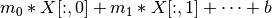.

Parameters: X : array, (M, D) The points to evaluate the model at. n : array of non-negative int, (D) The derivative order to take, specified as an integer order for each dimension in X. *args : num_dim+1 floats The slopes for each dimension, plus the constant term. Must be of the form m0, m1, ..., b.
class gptools.mean.LinearMeanFunction(num_dim=1, **kwargs)[source]

Linear mean function suitable for use with GaussianProcess.

Parameters: num_dim : positive int, optional The number of dimensions of the input data. Default is 1. **kwargs : optional kwargs All extra kwargs are passed to MeanFunction. If hyperprior and param_bounds are not specified, all parameters are taken to have a uniform hyperprior over [-1e3, 1e3].

## gptools.utils module¶

Provides convenient utilities for working with the classes and results from gptools.

class gptools.utils.JointPrior(i=1.0)[source]

Bases: object

Abstract class for objects implementing joint priors over hyperparameters.

In addition to the abstract methods defined in this template, implementations should also have an attribute named bounds which contains the bounds (for a prior with finite bounds) or the 95%% interval (for a prior which is unbounded in at least one direction).

__call__(theta, hyper_deriv=None)[source]

Evaluate the prior log-PDF at the given values of the hyperparameters, theta.

Parameters: theta : array-like, (num_params,) The hyperparameters to evaluate the log-PDF at. hyper_deriv : int or None, optional If present, return the derivative of the log-PDF with respect to the variable with this index.
random_draw(size=None)[source]

Draw random samples of the hyperparameters.

Parameters: size : None, int or array-like, optional The number/shape of samples to draw. If None, only one sample is returned. Default is None.
sample_u(q)[source]

Extract a sample from random variates uniform on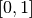.

For a univariate distribution, this is simply evaluating the inverse CDF. To facilitate efficient sampling, this function returns a vector of PPF values, one value for each variable. Basically, the idea is that, given a vector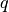of num_params values each of which is distributed uniformly on, this function will return corresponding samples for each variable.

Parameters: q : array-like, (num_params,) Values between 0 and 1 to evaluate inverse CDF at.
elementwise_cdf(p)[source]

Convert a sample to random variates uniform on.

For a univariate distribution, this is simply evaluating the CDF. To facilitate efficient sampling, this function returns a vector of CDF values, one value for each variable. Basically, the idea is that, given a vectorof num_params values each of which is distributed according to the prior, this function will return variables uniform oncorresponding to each variable. This is the inverse operation to sample_u().

Parameters: p : array-like, (num_params,) Values to evaluate CDF at.
__mul__(other)[source]

Multiply two JointPrior instances together.

class gptools.utils.CombinedBounds(l1, l2)[source]

Bases: object

Object to support reassignment of the bounds from a combined prior.

Works for any types of arrays.

Parameters: l1 : array-like The first list. l2 : array-like The second list.
__getitem__(pos)[source]

Get the item(s) at pos.

pos can be a basic slice object. But, the method is implemented by turning the internal array-like objects into lists, so only the basic indexing capabilities supported by the list data type can be used.

__setitem__(pos, value)[source]

Set the item at location pos to value.

Only works for scalar indices.

__len__()[source]

Get the length of the combined arrays.

__invert__()[source]

Return the elementwise inverse.

__str__()[source]

Get user-friendly string representation.

__repr__()[source]

Get exact string representation.

class gptools.utils.MaskedBounds(a, m)[source]

Bases: object

Object to support reassignment of free parameter bounds.

Parameters: a : array The array to be masked. m : array of int The indices in a which are to be accessible.
__getitem__(pos)[source]

Get the item(s) at location pos in the masked array.

__setitem__(pos, value)[source]

Set the item(s) at location pos in the masked array.

__len__()[source]

Get the length of the masked array.

__str__()[source]

Get user-friendly string representation.

__repr__()[source]

Get exact string representation.

class gptools.utils.ProductJointPrior(p1, p2)[source]

Product of two independent priors.

Parameters: p1, p2: :py:class:JointPrior instances The two priors to merge.
i
bounds
__call__(theta, hyper_deriv=None)[source]

Evaluate the prior log-PDF at the given values of the hyperparameters, theta.

The log-PDFs of the two priors are summed.

Parameters: theta : array-like, (num_params,) The hyperparameters to evaluate the log-PDF at.
sample_u(q)[source]

Extract a sample from random variates uniform on.

For a univariate distribution, this is simply evaluating the inverse CDF. To facilitate efficient sampling, this function returns a vector of PPF values, one value for each variable. Basically, the idea is that, given a vectorof num_params values each of which is distributed uniformly on, this function will return corresponding samples for each variable.

Parameters: q : array-like, (num_params,) Values between 0 and 1 to evaluate inverse CDF at.
elementwise_cdf(p)[source]

Convert a sample to random variates uniform on.

For a univariate distribution, this is simply evaluating the CDF. To facilitate efficient sampling, this function returns a vector of CDF values, one value for each variable. Basically, the idea is that, given a vectorof num_params values each of which is distributed according to the prior, this function will return variables uniform oncorresponding to each variable. This is the inverse operation to sample_u().

Parameters: p : array-like, (num_params,) Values to evaluate CDF at.
random_draw(size=None)[source]

Draw random samples of the hyperparameters.

The outputs of the two priors are stacked vertically.

Parameters: size : None, int or array-like, optional The number/shape of samples to draw. If None, only one sample is returned. Default is None.
class gptools.utils.UniformJointPrior(bounds, ub=None, **kwargs)[source]

Uniform prior over the specified bounds.

Parameters: bounds : list of tuples, (num_params,) The bounds for each of the random variables. ub : list of float, (num_params,), optional The upper bounds for each of the random variables. If present, bounds is then taken to be a list of float with the lower bounds. This gives UniformJointPrior a similar calling fingerprint as the other JointPrior classes.
__call__(theta, hyper_deriv=None)[source]

Evaluate the prior log-PDF at the given values of the hyperparameters, theta.

Parameters: theta : array-like, (num_params,) The hyperparameters to evaluate the log-PDF at.
sample_u(q)[source]

Extract a sample from random variates uniform on.

For a univariate distribution, this is simply evaluating the inverse CDF. To facilitate efficient sampling, this function returns a vector of PPF values, one value for each variable. Basically, the idea is that, given a vectorof num_params values each of which is distributed uniformly on, this function will return corresponding samples for each variable.

Parameters: q : array of float Values between 0 and 1 to evaluate inverse CDF at.
elementwise_cdf(p)[source]

Convert a sample to random variates uniform on.

For a univariate distribution, this is simply evaluating the CDF. To facilitate efficient sampling, this function returns a vector of CDF values, one value for each variable. Basically, the idea is that, given a vectorof num_params values each of which is distributed according to the prior, this function will return variables uniform oncorresponding to each variable. This is the inverse operation to sample_u().

Parameters: p : array-like, (num_params,) Values to evaluate CDF at.
random_draw(size=None)[source]

Draw random samples of the hyperparameters.

Parameters: size : None, int or array-like, optional The number/shape of samples to draw. If None, only one sample is returned. Default is None.
class gptools.utils.CoreEdgeJointPrior(bounds, ub=None, **kwargs)[source]

Prior for use with Gibbs kernel warping functions with an inequality constraint between the core and edge length scales.

__call__(theta, hyper_deriv=None)[source]

Evaluate the prior log-PDF at the given values of the hyperparameters, theta.

Parameters: theta : array-like, (num_params,) The hyperparameters to evaluate the log-PDF at.
sample_u(q)[source]

Extract a sample from random variates uniform on.

For a univariate distribution, this is simply evaluating the inverse CDF. To facilitate efficient sampling, this function returns a vector of PPF values, one value for each variable. Basically, the idea is that, given a vectorof num_params values each of which is distributed uniformly on, this function will return corresponding samples for each variable.

Parameters: q : array of float Values between 0 and 1 to evaluate inverse CDF at.
elementwise_cdf(p)[source]

Convert a sample to random variates uniform on.

For a univariate distribution, this is simply evaluating the CDF. To facilitate efficient sampling, this function returns a vector of CDF values, one value for each variable. Basically, the idea is that, given a vectorof num_params values each of which is distributed according to the prior, this function will return variables uniform oncorresponding to each variable. This is the inverse operation to sample_u().

Parameters: p : array-like, (num_params,) Values to evaluate CDF at.
random_draw(size=None)[source]

Draw random samples of the hyperparameters.

Parameters: size : None, int or array-like, optional The number/shape of samples to draw. If None, only one sample is returned. Default is None.
class gptools.utils.CoreMidEdgeJointPrior(bounds, ub=None, **kwargs)[source]

Prior for use with Gibbs kernel warping functions with an inequality constraint between the core, mid and edge length scales and the core-mid and mid-edge joins.

__call__(theta, hyper_deriv=None)[source]

Evaluate the prior log-PDF at the given values of the hyperparameters, theta.

Parameters: theta : array-like, (num_params,) The hyperparameters to evaluate the log-PDF at.
sample_u(q)[source]

Extract a sample from random variates uniform on.

For a univariate distribution, this is simply evaluating the inverse CDF. To facilitate efficient sampling, this function returns a vector of PPF values, one value for each variable. Basically, the idea is that, given a vectorof num_params values each of which is distributed uniformly on, this function will return corresponding samples for each variable.

Parameters: q : array of float Values between 0 and 1 to evaluate inverse CDF at.
elementwise_cdf(p)[source]

Convert a sample to random variates uniform on.

For a univariate distribution, this is simply evaluating the CDF. To facilitate efficient sampling, this function returns a vector of CDF values, one value for each variable. Basically, the idea is that, given a vectorof num_params values each of which is distributed according to the prior, this function will return variables uniform oncorresponding to each variable. This is the inverse operation to sample_u().

Parameters: p : array-like, (num_params,) Values to evaluate CDF at.
random_draw(size=None)[source]

Draw random samples of the hyperparameters.

Parameters: size : None, int or array-like, optional The number/shape of samples to draw. If None, only one sample is returned. Default is None.
class gptools.utils.IndependentJointPrior(univariate_priors)[source]

Joint prior for which each hyperparameter is independent.

Parameters: univariate_priors : list of callables or rv_frozen, (num_params,) The univariate priors for each hyperparameter. Entries in this list can either be a callable that takes as an argument the entire list of hyperparameters or a frozen instance of a distribution from scipy.stats.
__call__(theta, hyper_deriv=None)[source]

Evaluate the prior log-PDF at the given values of the hyperparameters, theta.

Parameters: theta : array-like, (num_params,) The hyperparameters to evaluate the log-PDF at.
bounds

The bounds of the random variable.

Set self.i=0.95 to return the 95% interval if this is used for setting bounds on optimizers/etc. where infinite bounds may not be useful.

sample_u(q)[source]

Extract a sample from random variates uniform on.

For a univariate distribution, this is simply evaluating the inverse CDF. To facilitate efficient sampling, this function returns a vector of PPF values, one value for each variable. Basically, the idea is that, given a vectorof num_params values each of which is distributed uniformly on, this function will return corresponding samples for each variable.

Parameters: q : array of float Values between 0 and 1 to evaluate inverse CDF at.
elementwise_cdf(p)[source]

Convert a sample to random variates uniform on.

For a univariate distribution, this is simply evaluating the CDF. To facilitate efficient sampling, this function returns a vector of CDF values, one value for each variable. Basically, the idea is that, given a vectorof num_params values each of which is distributed according to the prior, this function will return variables uniform oncorresponding to each variable. This is the inverse operation to sample_u().

Parameters: p : array-like, (num_params,) Values to evaluate CDF at.
random_draw(size=None)[source]

Draw random samples of the hyperparameters.

Parameters: size : None, int or array-like, optional The number/shape of samples to draw. If None, only one sample is returned. Default is None.
class gptools.utils.NormalJointPrior(mu, sigma, **kwargs)[source]

Joint prior for which each hyperparameter has a normal prior with fixed hyper-hyperparameters.

Parameters: mu : list of float, same size as sigma Means of the hyperparameters. sigma : list of float Standard deviations of the hyperparameters.
__call__(theta, hyper_deriv=None)[source]

Evaluate the prior log-PDF at the given values of the hyperparameters, theta.

Parameters: theta : array-like, (num_params,) The hyperparameters to evaluate the log-PDF at.
bounds

The bounds of the random variable.

Set self.i=0.95 to return the 95% interval if this is used for setting bounds on optimizers/etc. where infinite bounds may not be useful.

sample_u(q)[source]

Extract a sample from random variates uniform on.

For a univariate distribution, this is simply evaluating the inverse CDF. To facilitate efficient sampling, this function returns a vector of PPF values, one value for each variable. Basically, the idea is that, given a vectorof num_params values each of which is distributed uniformly on, this function will return corresponding samples for each variable.

Parameters: q : array of float Values between 0 and 1 to evaluate inverse CDF at.
elementwise_cdf(p)[source]

Convert a sample to random variates uniform on.

For a univariate distribution, this is simply evaluating the CDF. To facilitate efficient sampling, this function returns a vector of CDF values, one value for each variable. Basically, the idea is that, given a vectorof num_params values each of which is distributed according to the prior, this function will return variables uniform oncorresponding to each variable. This is the inverse operation to sample_u().

Parameters: p : array-like, (num_params,) Values to evaluate CDF at.
random_draw(size=None)[source]

Draw random samples of the hyperparameters.

Parameters: size : None, int or array-like, optional The number/shape of samples to draw. If None, only one sample is returned. Default is None.
class gptools.utils.LogNormalJointPrior(mu, sigma, **kwargs)[source]

Joint prior for which each hyperparameter has a log-normal prior with fixed hyper-hyperparameters.

Parameters: mu : list of float, same size as sigma Means of the logarithms of the hyperparameters. sigma : list of float Standard deviations of the logarithms of the hyperparameters.
__call__(theta, hyper_deriv=None)[source]

Evaluate the prior log-PDF at the given values of the hyperparameters, theta.

Parameters: theta : array-like, (num_params,) The hyperparameters to evaluate the log-PDF at.
bounds

The bounds of the random variable.

Set self.i=0.95 to return the 95% interval if this is used for setting bounds on optimizers/etc. where infinite bounds may not be useful.

sample_u(q)[source]

Extract a sample from random variates uniform on.

For a univariate distribution, this is simply evaluating the inverse CDF. To facilitate efficient sampling, this function returns a vector of PPF values, one value for each variable. Basically, the idea is that, given a vectorof num_params values each of which is distributed uniformly on, this function will return corresponding samples for each variable.

Parameters: q : array of float Values between 0 and 1 to evaluate inverse CDF at.
elementwise_cdf(p)[source]

Convert a sample to random variates uniform on.

For a univariate distribution, this is simply evaluating the CDF. To facilitate efficient sampling, this function returns a vector of CDF values, one value for each variable. Basically, the idea is that, given a vectorof num_params values each of which is distributed according to the prior, this function will return variables uniform oncorresponding to each variable. This is the inverse operation to sample_u().

Parameters: p : array-like, (num_params,) Values to evaluate CDF at.
random_draw(size=None)[source]

Draw random samples of the hyperparameters.

Parameters: size : None, int or array-like, optional The number/shape of samples to draw. If None, only one sample is returned. Default is None.
class gptools.utils.GammaJointPrior(a, b, **kwargs)[source]

Joint prior for which each hyperparameter has a gamma prior with fixed hyper-hyperparameters.

Parameters: a : list of float, same size as b Shape parameters. b : list of float Rate parameters.
__call__(theta, hyper_deriv=None)[source]

Evaluate the prior log-PDF at the given values of the hyperparameters, theta.

Parameters: theta : array-like, (num_params,) The hyperparameters to evaluate the log-PDF at.
bounds

The bounds of the random variable.

Set self.i=0.95 to return the 95% interval if this is used for setting bounds on optimizers/etc. where infinite bounds may not be useful.

sample_u(q)[source]

Extract a sample from random variates uniform on.

For a univariate distribution, this is simply evaluating the inverse CDF. To facilitate efficient sampling, this function returns a vector of PPF values, one value for each variable. Basically, the idea is that, given a vectorof num_params values each of which is distributed uniformly on, this function will return corresponding samples for each variable.

Parameters: q : array of float Values between 0 and 1 to evaluate inverse CDF at.
elementwise_cdf(p)[source]

Convert a sample to random variates uniform on.

For a univariate distribution, this is simply evaluating the CDF. To facilitate efficient sampling, this function returns a vector of CDF values, one value for each variable. Basically, the idea is that, given a vectorof num_params values each of which is distributed according to the prior, this function will return variables uniform oncorresponding to each variable. This is the inverse operation to sample_u().

Parameters: p : array-like, (num_params,) Values to evaluate CDF at.
random_draw(size=None)[source]

Draw random samples of the hyperparameters.

Parameters: size : None, int or array-like, optional The number/shape of samples to draw. If None, only one sample is returned. Default is None.
class gptools.utils.GammaJointPriorAlt(m, s, i=1.0)[source]

Joint prior for which each hyperparameter has a gamma prior with fixed hyper-hyperparameters.

This is an alternate form that lets you specify the mode and standard deviation instead of the shape and rate parameters.

Parameters: m : list of float, same size as s Modes s : list of float Standard deviations
a
b
class gptools.utils.SortedUniformJointPrior(num_var, lb, ub, **kwargs)[source]

Joint prior for a set of variables which must be strictly increasing but are otherwise uniformly-distributed.

Parameters: num_var : int The number of variables represented. lb : float The lower bound for all of the variables. ub : float The upper bound for all of the variables.
__call__(theta, hyper_deriv=None)[source]

Evaluate the log-probability of the variables.

Parameters: theta : array The parameters to find the log-probability of.
bounds
sample_u(q)[source]

Extract a sample from random variates uniform on.

For a univariate distribution, this is simply evaluating the inverse CDF. To facilitate efficient sampling, this function returns a vector of PPF values, one value for each variable. Basically, the idea is that, given a vectorof num_params values each of which is distributed uniformly on, this function will return corresponding samples for each variable.

Parameters: q : array of float Values between 0 and 1 to evaluate inverse CDF at.
elementwise_cdf(p)[source]

Convert a sample to random variates uniform on.

For a univariate distribution, this is simply evaluating the CDF. To facilitate efficient sampling, this function returns a vector of CDF values, one value for each variable. Basically, the idea is that, given a vectorof num_params values each of which is distributed according to the prior, this function will return variables uniform oncorresponding to each variable. This is the inverse operation to sample_u().

Parameters: p : array-like, (num_params,) Values to evaluate CDF at.
random_draw(size=None)[source]

Draw random samples of the hyperparameters.

Parameters: size : None, int or array-like, optional The number/shape of samples to draw. If None, only one sample is returned. Default is None.
gptools.utils.wrap_fmin_slsqp(fun, guess, opt_kwargs={})[source]

Wrapper for fmin_slsqp() to allow it to be called with minimize()-like syntax.

This is included to enable the code to run with scipy versions older than 0.11.0.

Accepts opt_kwargs in the same format as used by scipy.optimize.minimize(), with the additional precondition that the keyword method has already been removed by the calling code.

Parameters:

fun : callable

The function to minimize.

guess : sequence

The initial guess for the parameters.

opt_kwargs : dict, optional

Dictionary of extra keywords to pass to scipy.optimize.minimize(). Refer to that function’s docstring for valid options. The keywords ‘jac’, ‘hess’ and ‘hessp’ are ignored. Note that if you were planning to use jac = True (i.e., optimization function returns Jacobian) and have set args = (True,) to tell update_hyperparameters() to compute and return the Jacobian this may cause unexpected behavior. Default is: {}.

Returns:

Result : namedtuple

namedtuple that mimics the fields of the Result object returned by scipy.optimize.minimize(). Has the following fields:

 status int Code indicating the exit mode of the optimizer (imode from fmin_slsqp()) success bool Boolean indicating whether or not the optimizer thinks a minimum was found. fun float Value of the optimized function (-1*LL). x ndarray Optimal values of the hyperparameters. message str String describing the exit state (smode from fmin_slsqp()) nit int Number of iterations.
Raises:

ValueError

Invalid constraint type in constraints. (See documentation for scipy.optimize.minimize().)

gptools.utils.fixed_poch(a, n)[source]

Implementation of the Pochhammer symbol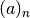which handles negative integer arguments properly.

Need conditional statement because scipy’s impelementation of the Pochhammer symbol is wrong for negative integer arguments. This function uses the definition from http://functions.wolfram.com/GammaBetaErf/Pochhammer/02/

Parameters: a : float The argument. n : nonnegative int The order.
gptools.utils.Kn2Der(nu, y, n=0)[source]

Find the derivatives of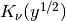.

Parameters: nu : float The order of the modified Bessel function of the second kind. y : array of float The values to evaluate at. n : nonnegative int, optional The order of derivative to take.
gptools.utils.yn2Kn2Der(nu, y, n=0, tol=0.0005, nterms=1, nu_step=0.001)[source]

Computes the function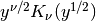and its derivatives.

Care has been taken to handle the conditions at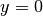.

For n=0, uses a direct evaluation of the expression, replacing points where y=0 with the appropriate value. For n>0, uses a general sum expression to evaluate the expression, and handles the value at y=0 using a power series expansion. Where it becomes infinite, the infinities will have the appropriate sign for a limit approaching zero from the right.

Uses a power series expansion aroundto avoid numerical issues.

Handles integer nu by performing a linear interpolation between values of nu slightly above and below the requested value.

Parameters: nu : float The order of the modified Bessel function and the exponent of y. y : array of float The points to evaluate the function at. These are assumed to be nonegative. n : nonnegative int, optional The order of derivative to take. Set to zero (the default) to get the value. tol : float, optional The distance from zero for which the power series is used. Default is 5e-4. nterms : int, optional The number of terms to include in the power series. Default is 1. nu_step : float, optional The amount to vary nu by when handling integer values of nu. Default is 0.001.
gptools.utils.incomplete_bell_poly(n, k, x)[source]

Recursive evaluation of the incomplete Bell polynomial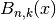.

Evaluates the incomplete Bell polynomial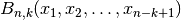, also known as the partial Bell polynomial or the Bell polynomial of the second kind. This polynomial is useful in the evaluation of (the univariate) Faa di Bruno’s formula which generalizes the chain rule to higher order derivatives.

The implementation here is based on the implementation in: sympy.functions.combinatorial.numbers.bell._bell_incomplete_poly() Following that function’s documentation, the polynomial is computed according to the recurrence formula: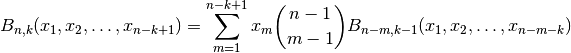The end cases are: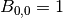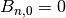for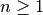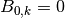for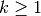Parameters: n : scalar int The first subscript of the polynomial. k : scalar int The second subscript of the polynomial. x : Array of floats, (p, n - k + 1) p sets of n - k + 1 points to use as the arguments to. The second dimension can be longer than required, in which case the extra entries are silently ignored (this facilitates recursion without needing to subset the array x). result : Array, (p,) Incomplete Bell polynomial evaluated at the desired values.
gptools.utils.generate_set_partition_strings(n)[source]

Generate the restricted growth strings for all of the partitions of an n-member set.

Uses Algorithm H from page 416 of volume 4A of Knuth’s The Art of Computer Programming. Returns the partitions in lexicographical order.

Parameters: n : scalar int, non-negative Number of (unique) elements in the set to be partitioned. partitions : list of Array List has a number of elements equal to the n-th Bell number (i.e., the number of partitions for a set of size n). Each element has length n, the elements of which are the restricted growth strings describing the partitions of the set. The strings are returned in lexicographic order.
gptools.utils.generate_set_partitions(set_)[source]

Generate all of the partitions of a set.

This is a helper function that utilizes the restricted growth strings from generate_set_partition_strings(). The partitions are returned in lexicographic order.

Parameters: set_ : Array or other Array-like, (m,) The set to find the partitions of. partitions : list of lists of Array The number of elements in the outer list is equal to the number of partitions, which is the len(m)^th Bell number. Each of the inner lists corresponds to a single possible partition. The length of an inner list is therefore equal to the number of blocks. Each of the arrays in an inner list is hence a block.
gptools.utils.powerset([1,2,3]) --> () (1,) (2,) (3,) (1,2) (1,3) (2,3) (1,2,3)[source]

From itertools documentation, https://docs.python.org/2/library/itertools.html.

gptools.utils.unique_rows(arr, return_index=False, return_inverse=False)[source]

Returns a copy of arr with duplicate rows removed.

From Stackoverflow “Find unique rows in numpy.array.”

Parameters: arr : Array, (m, n) The array to find the unique rows of. return_index : bool, optional If True, the indices of the unique rows in the array will also be returned. I.e., unique = arr[idx]. Default is False (don’t return indices). return_inverse: bool, optional If True, the indices in the unique array to reconstruct the original array will also be returned. I.e., arr = unique[inv]. Default is False (don’t return inverse). unique : Array, (p, n) where p <= m The array arr with duplicate rows removed.
gptools.utils.compute_stats(vals, check_nan=False, robust=False, axis=1, plot_QQ=False, bins=15, name='')[source]

Compute the average statistics (mean, std dev) for the given values.

Parameters: vals : array-like, (M, D) Values to compute the average statistics along the specified axis of. check_nan : bool, optional Whether or not to check for (and exclude) NaN’s. Default is False (do not attempt to handle NaN’s). robust : bool, optional Whether or not to use robust estimators (median for mean, IQR for standard deviation). Default is False (use non-robust estimators). axis : int, optional Axis to compute the statistics along. Presently only supported if robust is False. Default is 1. plot_QQ : bool, optional Whether or not a QQ plot and histogram should be drawn for each channel. Default is False (do not draw QQ plots). bins : int, optional Number of bins to use when plotting histogram (for plot_QQ=True). Default is 15 name : str, optional Name to put in the title of the QQ/histogram plot. mean : ndarray, (M,) Estimator for the mean of vals. std : ndarray, (M,) Estimator for the standard deviation of vals. NotImplementedError If axis != 1 when robust is True. NotImplementedError If plot_QQ is True.
gptools.utils.univariate_envelope_plot(x, mean, std, ax=None, base_alpha=0.375, envelopes=[1, 3], lb=None, ub=None, expansion=10, **kwargs)[source]

Make a plot of a mean curve with uncertainty envelopes.

gptools.utils.summarize_sampler(sampler, weights=None, burn=0, ci=0.95, chain_mask=None)[source]

Create summary statistics of the flattened chain of the sampler.

The confidence regions are computed from the quantiles of the data.

Parameters: sampler : emcee.Sampler instance or array, (n_temps, n_chains, n_samp, n_dim), (n_chains, n_samp, n_dim) or (n_samp, n_dim) The sampler to summarize the chains of. weights : array, (n_temps, n_chains, n_samp), (n_chains, n_samp) or (n_samp,), optional The weight for each sample. This is useful for post-processing the output from MultiNest sampling, for instance. burn : int, optional The number of samples to burn from the beginning of the chain. Default is 0 (no burn). ci : float, optional A number between 0 and 1 indicating the confidence region to compute. Default is 0.95 (return upper and lower bounds of the 95% confidence interval). chain_mask : (index) array, optional Mask identifying the chains to keep before plotting, in case there are bad chains. Default is to use all chains. mean : array, (num_params,) Mean values of each of the parameters sampled. ci_l : array, (num_params,) Lower bounds of the ci*100% confidence intervals. ci_u : array, (num_params,) Upper bounds of the ci*100% confidence intervals.
gptools.utils.plot_sampler(sampler, weights=None, cutoff_weight=None, labels=None, burn=0, chain_mask=None, bins=50, points=None, covs=None, colors=None, ci=[0.95], plot_samples=False, plot_hist=True, chain_alpha=0.1, temp_idx=0, label_fontsize=14, ticklabel_fontsize=9, chain_label_fontsize=9, chain_ticklabel_fontsize=7, suptitle=None, bottom_sep=0.075, label_chain_y=False, max_chain_ticks=6, max_hist_ticks=None, chain_ytick_pad=2.0, suptitle_space=0.1, fixed_width=None, fixed_height=None, ax_space=0.1, cmap='gray_r', hide_chain_ylabels=False, plot_chains=True)[source]

Plot the results of MCMC sampler (posterior and chains).

Loosely based on triangle.py. Provides extensive options to format the plot.

Parameters: sampler : emcee.Sampler instance or array, (n_temps, n_chains, n_samp, n_dim), (n_chains, n_samp, n_dim) or (n_samp, n_dim) The sampler to plot the chains/marginals of. Can also be an array of samples which matches the shape of the chain attribute that would be present in a emcee.Sampler instance. weights : array, (n_temps, n_chains, n_samp), (n_chains, n_samp) or (n_samp,), optional The weight for each sample. This is useful for post-processing the output from MultiNest sampling, for instance. cutoff_weight : float, optional If weights and cutoff_weight are present, points with weights < cutoff_weight * weights.max() will be excluded. Default is to plot all points. labels : list of str, optional The labels to use for each of the free parameters. Default is to leave the axes unlabeled. burn : int, optional The number of samples to burn before making the marginal histograms. Default is zero (use all samples). chain_mask : (index) array, optional Mask identifying the chains to keep before plotting, in case there are bad chains. Default is to use all chains. points : array, (D,) or (N, D), optional Array of point(s) to plot onto each marginal and chain. Default is None. covs : array, (D, D) or (N, D, D), optional Covariance matrix or array of covariance matrices to plot onto each marginal. If you do not want to plot a covariance matrix for a specific point, set its corresponding entry to None. Default is to not plot confidence ellipses for any points. ci : array, (num_ci,), optional List of confidence intervals to plot for each non-None entry in covs. Default is 0.95 (just plot the 95 percent confidence interval). plot_samples : bool, optional If True, the samples are plotted as individual points. Default is False. chain_alpha : float, optional The transparency to use for the plots of the individual chains. Setting this to something low lets you better visualize what is going on. Default is 0.1. temp_idx : int, optional Index of the temperature to plot when plotting a emcee.PTSampler. Default is 0 (samples from the posterior). label_fontsize : float, optional The font size (in points) to use for the axis labels. Default is 16. ticklabel_fontsize : float, optional The font size (in points) to use for the axis tick labels. Default is 10. chain_label_fontsize : float, optional The font size (in points) to use for the labels of the chain axes. Default is 10. chain_ticklabel_fontsize : float, optional The font size (in points) to use for the chain axis tick labels. Default is 6. suptitle : str, optional The figure title to place at the top. Default is no title. bottom_sep : float, optional The separation (in relative figure units) between the chains and the marginals. Default is 0.075. label_chain_y : bool, optional If True, the chain plots will have y axis labels. Default is False. max_chain_ticks : int, optional The maximum number of y-axis ticks for the chain plots. Default is 6. max_hist_ticks : int, optional The maximum number of ticks for the histogram plots. Default is None (no limit). chain_ytick_pad : float, optional The padding (in points) between the y-axis tick labels and the axis for the chain plots. Default is 2.0. suptitle_space : float, optional The amount of space (in relative figure units) to leave for a figure title. Default is 0.1. fixed_width : float, optional The desired figure width (in inches). Conflicts with fixed_height. fixed_height : float, optional The desired figure height (in inches). Conflicts with fixed_width. ax_space : float, optional The w_space and h_space to use. Default is 0.1. plot_chains : bool, optional If True, plot the sampler chains. Default is True.
gptools.utils.plot_sampler_fingerprint(sampler, hyperprior, weights=None, cutoff_weight=None, nbins=None, labels=None, burn=0, chain_mask=None, temp_idx=0, points=None, plot_samples=False, sample_color='k', point_color=None, point_lw=3, title='', rot_x_labels=False, figsize=None)[source]

Make a plot of the sampler’s “fingerprint”: univariate marginal histograms for all hyperparameters.

The hyperparameters are mapped to [0, 1] using hyperprior.elementwise_cdf(), so this can only be used with prior distributions which implement this function.

Returns the figure and axis created.

Parameters: sampler : emcee.Sampler instance or array, (n_temps, n_chains, n_samp, n_dim), (n_chains, n_samp, n_dim) or (n_samp, n_dim) The sampler to plot the chains/marginals of. Can also be an array of samples which matches the shape of the chain attribute that would be present in a emcee.Sampler instance. hyperprior : JointPrior instance The joint prior distribution for the hyperparameters. Used to map the values to [0, 1] so that the hyperparameters can all be shown on the same axis. weights : array, (n_temps, n_chains, n_samp), (n_chains, n_samp) or (n_samp,), optional The weight for each sample. This is useful for post-processing the output from MultiNest sampling, for instance. cutoff_weight : float, optional If weights and cutoff_weight are present, points with weights < cutoff_weight * weights.max() will be excluded. Default is to plot all points. nbins : int or array of int, (D,), optional The number of bins dividing [0, 1] to use for each histogram. If a single int is given, this is used for all of the hyperparameters. If an array of ints is given, these are the numbers of bins for each of the hyperparameters. The default is to determine the number of bins using the Freedman-Diaconis rule. labels : array of str, (D,), optional The labels for each hyperparameter. Default is to use empty strings. burn : int, optional The number of samples to burn before making the marginal histograms. Default is zero (use all samples). chain_mask : (index) array, optional Mask identifying the chains to keep before plotting, in case there are bad chains. Default is to use all chains. temp_idx : int, optional Index of the temperature to plot when plotting a emcee.PTSampler. Default is 0 (samples from the posterior). points : array, (D,) or (N, D), optional Array of point(s) to plot as horizontal lines. Default is None. plot_samples : bool, optional If True, the samples are plotted as horizontal lines. Default is False. sample_color : str, optional The color to plot the samples in. Default is ‘k’, meaning black. point_color : str or list of str, optional The color to plot the individual points in. Default is to loop through matplotlib’s default color sequence. If a list is provided, it will be cycled through. point_lw : float, optional Line width to use when plotting the individual points. title : str, optional Title to use for the plot. rot_x_labels : bool, optional If True, the labels for the x-axis are rotated 90 degrees. Default is False (do not rotate labels). figsize : 2-tuple, optional The figure size to use. Default is to use the matplotlib default.
gptools.utils.plot_sampler_cov(sampler, method='corr', weights=None, cutoff_weight=None, labels=None, burn=0, chain_mask=None, temp_idx=0, cbar_label=None, title='', rot_x_labels=False, figsize=None, xlabel_on_top=True)[source]

Make a plot of the sampler’s correlation or covariance matrix.

Returns the figure and axis created.

Parameters: sampler : emcee.Sampler instance or array, (n_temps, n_chains, n_samp, n_dim), (n_chains, n_samp, n_dim) or (n_samp, n_dim) The sampler to plot the chains/marginals of. Can also be an array of samples which matches the shape of the chain attribute that would be present in a emcee.Sampler instance. method : {‘corr’, ‘cov’} Whether to plot the correlation matrix (‘corr’) or the covariance matrix (‘cov’). The covariance matrix is often not useful because different parameters have wildly different scales. Default is to plot the correlation matrix. labels : array of str, (D,), optional The labels for each hyperparameter. Default is to use empty strings. burn : int, optional The number of samples to burn before making the marginal histograms. Default is zero (use all samples). chain_mask : (index) array, optional Mask identifying the chains to keep before plotting, in case there are bad chains. Default is to use all chains. temp_idx : int, optional Index of the temperature to plot when plotting a emcee.PTSampler. Default is 0 (samples from the posterior). cbar_label : str, optional The label to use for the colorbar. The default is chosen based on the value of the method keyword. title : str, optional Title to use for the plot. rot_x_labels : bool, optional If True, the labels for the x-axis are rotated 90 degrees. Default is False (do not rotate labels). figsize : 2-tuple, optional The figure size to use. Default is to use the matplotlib default. xlabel_on_top : bool, optional If True, the x-axis labels are put on top (the way mathematicians present matrices). Default is True.

## Module contents¶

gptools - Gaussian process regression with support for arbitrary derivatives